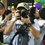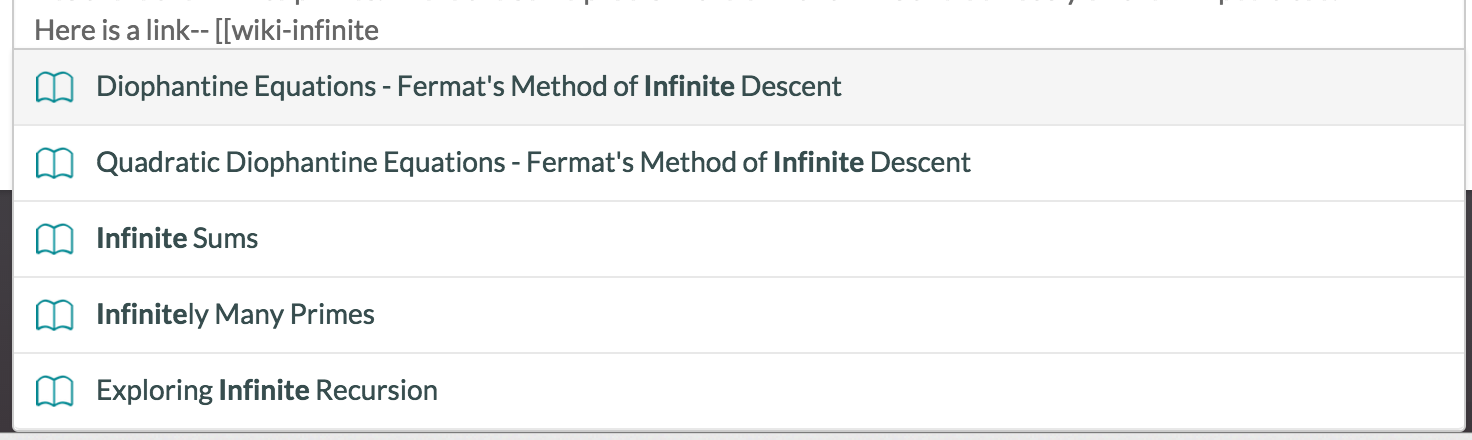Are there infinite primes? If yes, or no, could you prove it? Any useful internet resources?

Is there a usual pattern for primes?

How to define Mersenne primes? ( $M_n=2^n-1)$. And how many of them had been found?

And others? Like Wilson's Theorem $(n-1)! \equiv -1 (\text{mod n})$? Or all coefficients of $(x-1)^y -(y^p)-1$ is divisible by $y$, then $y$ must be prime.

Recently, I knew that the largest known prime number, which is discovered in August $2015$ by Great Internet Mersenne Prime Search, is $\displaystyle \Huge{ \color{#20A900}{2}^{\color{#3D99F6}{57,885,161}} - \color{#D61F06}{1}}$It contains $17, 425,170$ digits! It's a Mersene prime.

Also, the largest non-Mersenne prime number is:

$\Huge \color{#20A900}{19249} \color{#D61F06}{\times 2^{\color{#3D99F6}{13018586}}} + 1$5 years, 11 months ago

This discussion board is a place to discuss our Daily Challenges and the math and science related to those challenges. Explanations are more than just a solution — they should explain the steps and thinking strategies that you used to obtain the solution. Comments should further the discussion of math and science.

When posting on Brilliant:

• Use the emojis to react to an explanation, whether you're congratulating a job well done , or just really confused .
• Ask specific questions about the challenge or the steps in somebody's explanation. Well-posed questions can add a lot to the discussion, but posting "I don't understand!" doesn't help anyone.
• Try to contribute something new to the discussion, whether it is an extension, generalization or other idea related to the challenge.

MarkdownAppears as
*italics* or _italics_ italics
**bold** or __bold__ bold
- bulleted- list
• bulleted
• list
1. numbered2. list
1. numbered
2. list
Note: you must add a full line of space before and after lists for them to show up correctly
paragraph 1paragraph 2

paragraph 1

paragraph 2

[example link](https://brilliant.org)example link
> This is a quote
This is a quote
    # I indented these lines
# 4 spaces, and now they show
# up as a code block.

print "hello world"
# I indented these lines
# 4 spaces, and now they show
# up as a code block.

print "hello world"
MathAppears as
Remember to wrap math in $$ ... $$ or $ ... $ to ensure proper formatting.
2 \times 3 $2 \times 3$
2^{34} $2^{34}$
a_{i-1} $a_{i-1}$
\frac{2}{3} $\frac{2}{3}$
\sqrt{2} $\sqrt{2}$
\sum_{i=1}^3 $\sum_{i=1}^3$
\sin \theta $\sin \theta$
\boxed{123} $\boxed{123}$

Sort by:

I was excited to hear they had discovered a new larger Mersenne prime. Then I read closer and found that this is the largest known prime so far as of this month.

It was actually discovered back in 2013. Seems like it's past time they found a bigger one, right?

- 5 years, 11 months ago

Yes there are infinite primes. There are some proofs in the brilliant wikis and obviously on the wikipedia too. Here is a link-- Infinitely Many Primes

- 5 years, 11 months ago

FYI, you can use our nifty wiki linking tool to easily link to wiki pagesStaff - 5 years, 11 months ago

understood. Thanks.

- 5 years, 11 months ago

Nope, there is no pattern to primes, in the sense that there's no simple formula that we can plug numbers into to calculate the nth prime.

However, there are some cool patterns relating to the distribution of primes among the integers. I vaguely remember a nice YouTube video about that: I'll link it here if I can find it...

- 5 years, 11 months ago